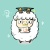0+0+0+0+0+0+0+0+0+0+0+0+0+0+0+Tensor networks have found a wide use in a variety of applications in physics and computer science, recently leading to both theoretical insights as well as practical algorithms in machine learning. In this work we explore the connection between tensor networks and probabilistic graphical models, and show that it motivates the definition of generalized tensor networks where information from a tensor can be copied and reused in other parts of the network. We discuss the relationship between generalized tensor network architectures used in quantum physics, such as string-bond states, and architectures commonly used in machine learning. We provide an algorithm to train these networks in a supervised-learning context and show that they overcome the limitations of regular tensor networks in higher dimensions, while keeping the computation efficient. A method to combine neural networks and tensor networks as part of a common deep learning architecture is also introduced. We benchmark our algorithm for several generalized tensor network architectures on the task of classifying images and sounds, and show that they outperform previously introduced tensor-network algorithms. The models we consider also have a natural implementation on a quantum computer and may guide the development of near-term quantum machine learning architectures.0+0+0+下载预览Abstraction reasoning is a long-standing challenge in artificial intelligence. Recent studies suggest that many of the deep architectures that have triumphed over other domains failed to work well in abstract reasoning. In this paper, we first illustrate that one of the main challenges in such a reasoning task is the presence of distracting features, which requires the learning algorithm to leverage counterevidence and to reject any of the false hypotheses in order to learn the true patterns. We later show that carefully designed learning trajectory over different categories of training data can effectively boost learning performance by mitigating the impacts of distracting features. Inspired by this fact, we propose feature robust abstract reasoning (FRAR) model, which consists of a reinforcement learning based teacher network to determine the sequence of training and a student network for predictions. Experimental results demonstrated strong improvements over baseline algorithms and we are able to beat the state-of-the-art models by 18.7% in the RAVEN dataset and 13.3% in the PGM dataset.0+0+0+下载预览0+0+0+Learning general latent-variable probabilistic graphical models is a key theoretical challenge in machine learning and artificial intelligence. All previous methods, including the EM algorithm and the spectral algorithms, face severe limitations that largely restrict their applicability and affect their performance. In order to overcome these limitations, in this paper we introduce a novel formulation of message-passing inference over junction trees named predictive belief propagation, and propose a new learning and inference algorithm for general latent-variable graphical models based on this formulation. Our proposed algorithm reduces the hard parameter learning problem into a sequence of supervised learning problems, and unifies the learning of different kinds of latent graphical models into a single learning framework, which is local-optima-free and statistically consistent. We then give a proof of the correctness of our algorithm and show in experiments on both synthetic and real datasets that our algorithm significantly outperforms both the EM algorithm and the spectral algorithm while also being orders of magnitude faster to compute.0+0+0+下载预览0+1+0+0+0+0+0+0+0+0+0+0+0+0+0+0+0+0+
Top